blog

# Ultimate Guide To The Synthetic Long Stock Strategy

#### Options Trading 101 - The Ultimate Beginners Guide To Options

As Seen On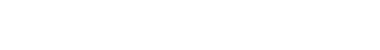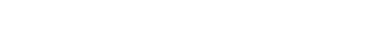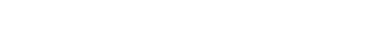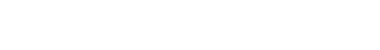by Gavin in BlogToday, we’re looking at the synthetic long stock strategy.

A synthetic long stock is a means of recreating the payoff profile of a long stock using options.

It is a combination of a long call and short put.

## Introduction

A synthetic long stock is a means of recreating the payoff profile of a long stock using options.

It is a combination of a long call and short put on the same underlying stock with identical strike price and expiration.

Simply put, a synthetic long stock position uses options to replicate the payoff of holding 100 shares of the stock without actually owning it.

This results in a leveraged position as the margin requirements for a synthetic long are much less than buying 100 shares.

It would be unfair if I just state the definition without providing an understanding of the building block of synthetic positions – known as the put-call parity.

Put-call parity shows the position equivalence of a portfolio of a call and risk-free bond with a portfolio of underlying stock and a put.

It defines the relationship between call prices and put prices with identical strike price and expiration.

In mathematical terms, put-call parity states:

C + X/(1+r)^t = S + P.

Where,

C = Call

X = Risk free bond

S = Stock

P = Put

Basically, it means that the price of call option has some information about the price of the corresponding put option, and vice versa.

I could spend an entire month talking about the derivation of put-call parity formula, but essentially the parity holds because any material divergence between put and call values will correct themselves as arbitrageurs take advantage of the mispricing.

It only holds for options that are held to expiration, but we don’t have to worry about that for now.

A simple understanding of the parity is enough to understand synthetic positions.

For the purposes of understanding synthetics, let’s assume interest rates are zero and the stock doesn’t pay a dividend.

Ok, so now that we have that out of the way. Let’s revisit the put-call parity and understand the synthetic positions formulaically and intuitively.

Formulaically if we rearrange the parity formula from above such that you have long stock on one side and the rest on the other side.

S = C – P – X(1+r)^t

We can ignore the risk-free bond (X) because all it means is that you have the money in your account to meet the strike price at expiration. Therefore, restated it is:

S = C – P (i.e. long stock equals long call and short put)

Formulas are fun but let’s understand the relationship intuitively.

Remember the payoff of a long stock. It states the value of your position increases as stock price rises above the purchase price and it decreases as stock price falls below the purchase price.

A synthetic strategy simply asks: How can we replicate the same payoff without buying the stock?

We can do this by using options.

If we buy a call option with a strike price of x (assume x equals purchase price of stock), the payoff will increase as the stock price rises above x, but will flatline as the stock price falls below x.

On the other hand, if we sell a put with the same strike price of x, the payoff will decrease as the stock price falls below x, but will flatline as the stock price rises above x.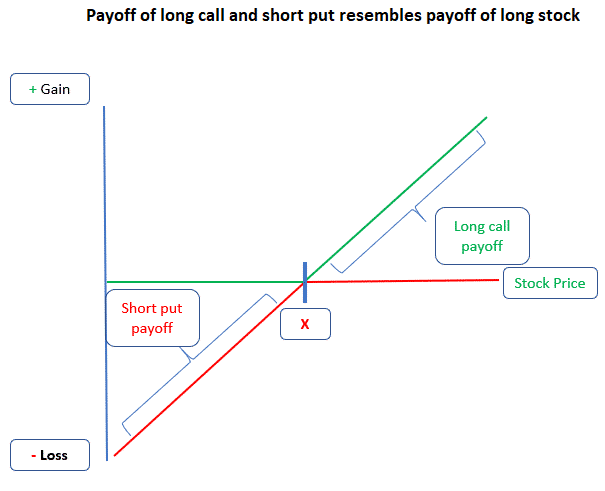This assumed there was no net debit or credit to enter the trade, and that strike price on options equaled purchase price of the stock. We will relax these assumptions later.

Side note, when I first learned about put-call parity, I had the hardest time getting the equation straight in my head so a mnemonic I found helpful was:

Cow Boy Sings Pretty → Call + Bond = Stock + Put

Hopefully that comes in handy!

Let’s introduce an example of how the synthetic long looks and this is the example we will use for the next few sections.

An investor wants to synthetically create the same payoff as a long stock of Uber when it is trading at \$34.69.

Unfortunately, there is no option with the same strike as the stock price so in this example, the investor selects the long call that is slightly in the money.

If on expiration, the stock is above the strike price of \$34.5, the investor can exercise the call and buy the shares at \$34.5.

If on expiration, the stock is below the strike price of \$34.5, the put will be exercised and the investor will be forced to buy the shares at \$34.5

Either way, if you hold the synthetic, you will end up buying the stock.

So, why not just buy the stock upfront? As mentioned earlier, a synthetic long is a leveraged position, so the investor can trade more positions for the same amount of capital.

Of course, leverage is a two-edged sword and if the stock falls, losses will be magnified.

Below is the payoff diagram for the Uber synthetic long which we will use as our example for today’s article.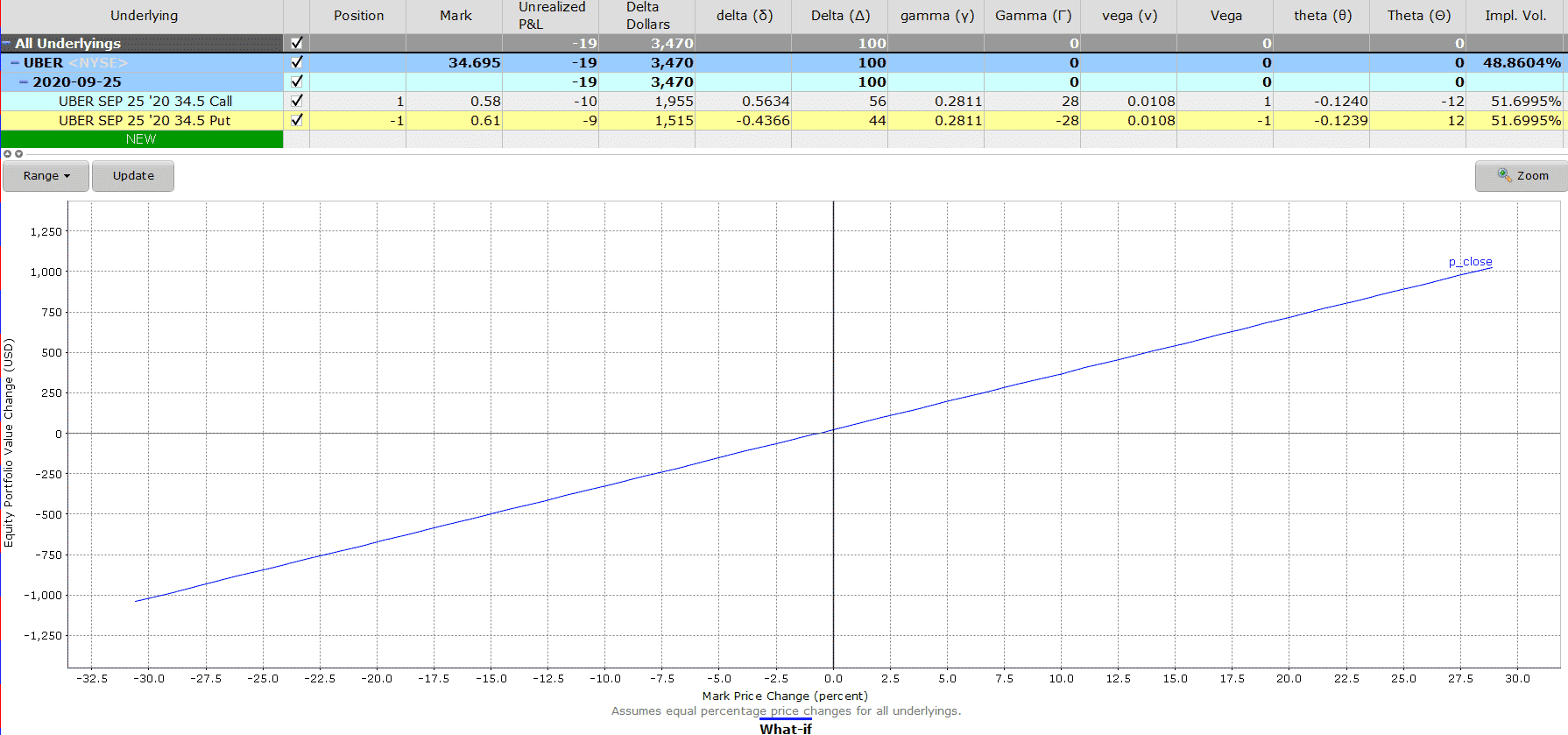## Maximum Loss

A long synthetic stock is replicating the payoff of the stock. So the maximum loss will equal the maximum loss if you were simply long a stock.

You stand to lose the strike price on the put. If the stock falls to zero, the put will be assigned to you at \$34.5. Your maximum loss will be \$34.5 x 100 = \$3,450.

But wait, you received a payment of \$0.58 to enter the long position, and received proceeds of \$0.61 for the short put, so you had a net credit of \$0.03.

Thus, your maximum loss is slightly lower thanks to the net credit received i.e. \$34.47 x 100 = \$3,447.

## Maximum Gain

Technically the stock could rise to infinity, but most people don’t want to wait until expiration and be assigned the stock.

Remember, if you wanted to buy the stock outright, you could have just bought it in the market.

The maximum gain will depend on the price of the stock moments before expiration.

Time value is essentially zero by then so the value of your short put is zero, and the value of your long call will be price of the stock minus call strike.

Let’s assume Uber is trading at \$36 right before expiration, and you exit out of the long synthetic – your gain will be \$1.5 (\$36 – \$34.5)

Remember to add the net credit received when entering the synthetic position.

## Breakeven Price

We received a net credit of \$0.03 to enter the synthetic position.

We will break even if the stock at expiration falls from strike of \$34.5 to \$34.47.

More generally, the breakeven is strike + net debit paid, or strike – net credit received.

## Payoff Diagram

The potential profit is unlimited, and the potential losses are significant.

As you can see the payoff diagram very closely resembles the long stock payoff.## The Greeks

### Delta

A synthetic long stock position has two options. A long call and a short put.

Both options are at the money so the call delta should be close to +0.5 and the put delta should be close to -0.5.

But remember, we couldn’t find options with the strike price equal to the current price.

The call we selected is slightly in the money with a delta of 0.56, and the put we shorted has a delta of 0.43.

In total, the delta of our position is 0.99 – close to the delta of long stock of 1.

In other words, our synthetic long stock position will behave just like a long stock would.

It would change by 0.99 for every \$1 change in the stock.

### Gamma

Gamma measures the rate of change in delta with respect to the change in stock price.

When delta is at its highest and can no longer change much, the rate of change in delta by definition goes to zero.

We know that call delta ranges between 0 and 1.

Deep out of money calls have a delta of 0, at the money calls have a delta of 0.5, and deep in the money calls have a delta of 1.

If gamma measures the change in delta with respect to the change in stock price, then at the money options will be highly susceptible to changes in the stock price. Therefore, gamma of at the money calls are the highest.

The long call in our example has a gamma of 0.28.

The short put in our example has a gamma of -0.28.

The two offset each other, therfore gamma has no impact on the synthetic long stock position.

## How Volatility Impacts the Trade

Long call options are long vega trades. They will benefit if volatility rises after the trade has been placed.

Short put options are short vega trades. They will be at a disadvantage if volatility rises after the trade has been placed.

The long call has a vega of 0.0108 i.e. the value of the long call option will increase by \$0.0108 if implied volatility increases by one point.

The short put has a vega of -0.0108 i.e. the value of the short put option will decrease by \$0.0108 if implied volatility increases by one point.

When implementing a synthetic long stock, the long call’s volatility offsets the short put’s volatility.

## How Theta Impacts the Trade

Theta measures how sensitive the option price is to the passage of time – i.e. how much value does the option win/lose each day as the trade approaches expiration.

We are long a call and short a put with the same strike and expiration, so loss of value in long call option will be offset by the gain in value in short put option.

The long call will lose by 20.6% with each passing day (theta of (12) / call price (\$58)).

The short put will gain by 19.6% with each passing day (theta of (12) / call price (\$61)).

Like the other greeks, theta will have very little impact on a synthetic long position.

## Risks

Just like owning the stock, the risk of owning a synthetic stock is that stock price can tank.

However, unlike owning the stock, the risks here are magnified because you have levered up your portfolio using options.

Even though options are generally not assigned until expiration, there is a risk of early assignment of the short put if it goes deep in the money before expiration.

## Example

Date: Aug 6, 2020

Price: \$34.70

Buy one Sep 25 UBER \$34.5 call @ \$0.58
Sell one Sep 25 UBER \$34.5 put @ \$0.61

Net debit: \$2

This simulates a stock position.

Therefore, the investor manages the position like a stock.

On September 11, the price of UBER is at the top of upper Bollinger Band and RSI at 70 heading into over-bought condition.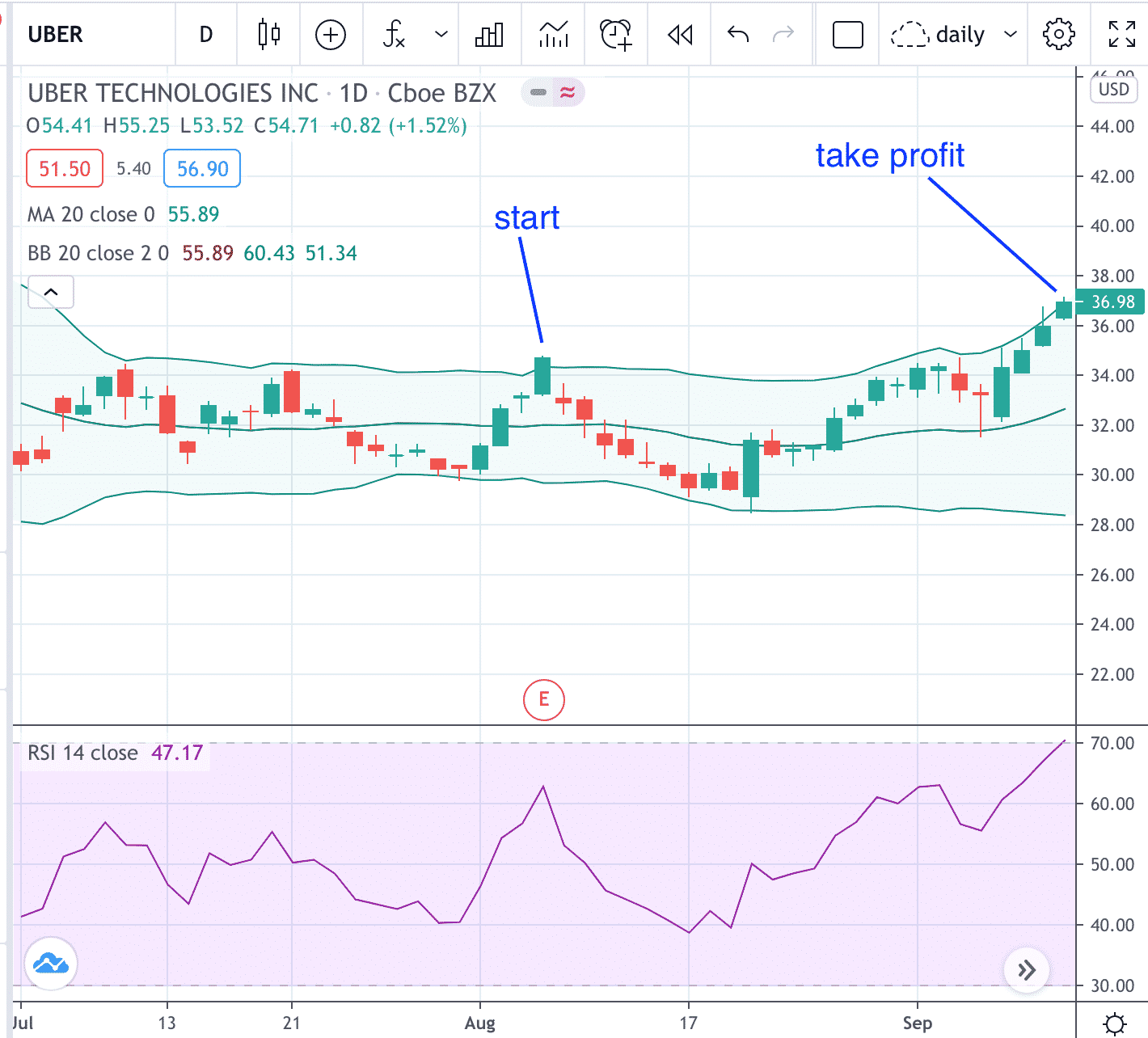The investor understands that there are only two more weeks before the synthetic position expires and decides to close the position to take profit.

Date: Sept 11

Price: \$36.99

Buy to close one Sep 25 UBER \$34.5 put @ \$0.48
Sell to close one Sep 25 UBER \$34.5 call @ \$2.96

Credit: \$248

Considering that the investor paid \$2 to initiate the synthetic, the net profit for the investor is \$246.

This is calculated as \$248 – \$2 = \$246

Note that the long call gained a lot of value while the short put lost some value.

How does that compare with the investor who had purchased 100 shares of UBER instead?

\$36.99 – \$34.70 = \$2.29 per share

Profit for the stock investor: \$229

The stock investor had about \$3500 of capital tied up in the UBER position.

In an IRA account, the option investor would need to put aside about \$3450 in cash for the cash-secured put in the event that the short put is assigned and that the investor would actually need to purchase the 100 shares of UBER.

## Summary

A synthetic long stock is a way to recreate the payoff of a stock using options.

Be wary because if you don’t exit out of the option positions before expiration, you may end up with the stock even if you did not want to.

A synthetic long stock strategy is a leveraged position and traders need to be aware the gains and losses will be magnified in percentage terms.